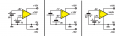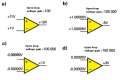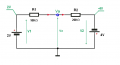# operational amplifier and measuring voltage drop

#### logearav

Joined Aug 19, 2011
243
Dear Members,
I have attached the image of inverting operational amplifier. I have the following doubts
For measuring current input current Iin we take voltage drop across Ri as
Vin - Va
Similarly voltage drop across Rf as Va - Vout for the current If
My question is why not we take as Va - Vin, for the first case and
Vout - Va for the second case

#### Jony130

Joined Feb 17, 2009
5,457
•logearav

#### steveb

Joined Jul 3, 2008
2,436
My question is why not we take as Va - Vin, for the first case and
Vout - Va for the second case
You can do that if you like. You are free to define the voltage drops and associated current directions arbitrarily. However, once you define them, you have to correctly apply Kirchoff's Voltage Law and Kirchoff's Current Law using those definitions.

The current flows from left to right in this diagram.
I agree with Jony130. Once you make this statement, you have effectively defined the direction and the polarity, and your formulas are a consequence of this decision.

•logearav

#### logearav

Joined Aug 19, 2011
243
Thanks for the reply Mr. Jony130 and Mr. Steveb.
Mr. Jony, you said Va is at higher potential than Vin and similarly Va is at higher potential than Vout. But in your diagram a) Vin is -1 volt and Va is 0 Volt. Does this mean -1 is greater than O volt. Also Vout is 2 volt. Vout is higher than Va but how
0 ( Va) > 2 ( Vout)?

#### Jony130

Joined Feb 17, 2009
5,457
But in your diagram a) Vin is -1 volt and Va is 0 Volt. Does this mean -1 is greater than O volt. Also Vout is 2 volt. Vout is higher than Va but how
0 ( Va) > 2 ( Vout)?
Diagram a) show the situation when the current flow from "right to left".
So in this case we have Vout>Va and Va>Vin

And diagram b) show the current flows from "left to right".

Simply, the opamp output attempts to do whatever is necessary to make the voltage difference between the inputs zero.
So if you have 0V at the "+" input and the the opamps wants to have the same voltage at it's "-" input (0V) the only way to do this is by increasing it's output voltage to 2V if input voltage is -1V.
And for case b) to make the voltage difference between the inputs equal to zero. The opamp will decrease it's output voltage to -4V.
Because only when the output voltage reach -4V the difference between the "+" input and "-" input is equal to 0V for +2V input voltage.

•logearav

#### logearav

Joined Aug 19, 2011
243
Thanks John130. " the op amp attempts to do whatever is necessary to make the voltage difference between the inputs zero"
1)Does this apply only to inverting amplifier alone or all types of amplifier, say non inverting amp, summing amp, difference amp etc?
2) How the voltage becomes 0 at the junction between the two resistors R1 and R2?

#### Jony130

Joined Feb 17, 2009
5,457
Thanks John130. " the op amp attempts to do whatever is necessary to make the voltage difference between the inputs zero"
1)Does this apply only to inverting amplifier alone or all types of amplifier, say non inverting amp, summing amp, difference amp etc?
This is general case rule apply for all op amp circuit.
And we call it "virtual short" ( linear region)

Sometimes when op amp was unable to "bring" inputs to zero. The op amp will entering the saturation mode. The output voltage is equal the power supply voltage.In real life the op amp alone (without any feedback loop) is nothing more then a differential amplifier.
The output voltage is the difference between the + and - inputs multiplied by the open-loop gain:
Vout = (V"+" − V"−")*G_open_loop.So when the voltage at the input "+" (non-inverting) rise,output voltage is also increases.
Increase voltage at "-" input (inverting input) causes, decrease the output voltage.
Decrease voltage at input "-" increases the output voltage.But in circuit theory we assume that the open loop gain is infinitely large.
And we add the feedback network.
So for example in case a) we apply 1V into the input terminal of a Non-inverting amplifier. The op amp output voltage will start rising because we have 1V at V"+" input and 0V at V"-".
So the output voltage rise until non-inverting input voltages (+) will be equal to inverting input voltages (-)We have similar situation in Inverting amplifier.For example in case b)
We apply 2V at inverting input so the op amp see the potential difference between his inputs. So the output voltage start to starts to decrease. And it will be decrease until V"+" = V"-". As you can see nothing magical is happening here.
When we apply positive voltage at inverting input, the op amp output voltage start to decrease.
But thanks to R2 some part of the output voltage is feedback to inverting input.
And when V"+" = V"-" the op amp see 0V difference between his inputs terminals.
And output voltage stop changing.
From the viewer perspective op amp "magically" brought his input terminal differential voltages to 0.

The more detailed explanation
Now let us understand how the negative feedback returned through R2 affects the amplifier operation. To begin our discussion, let us momentarily freeze the input signal as it passes through 0 volts. At this instant, the op amp has no
input voltage (i.e., VD = 0 = voltage between the (+) and (-) input terminals (VD)). It is this differential input voltage that is amplified by the gain of the op amp to become the output voltage. In this case, the output voltage will be 0. Now suppose the output voltage tried to drift in a positive direction. Can you see that this positive change would be felt through RF and would cause the inverting pin (-) of the op amp to become slightly positive Since essentially no current flows in or out of the op amp input and the (+) input of the op amp is at ground potential. This causes VD to be greater than 0 with the (-) terminal being the most positive. When VD is amplified by the op amp it appears in the output as a negative voltage (inverting
amplifier action). This forces the output, which had initially tried to drift in a positive
direction, to return to its 0 state. A similar, but opposite, action would occur if the output tried to drift in the negative direction. Thus, as long as the input is held at 0 volts, the output is forced to stay at 0 volts.
Now suppose we allow the input signal to rise to a +2 volt instantaneous level and freeze it for purposes of the following discussion. With +2 volts applied to R1 and 0 at the output of the op amp, the voltage divider made up of R2 and R1 will have two volts across it. Since the (-) terminal of the op amp does not draw any significant current, the voltage divider is essentially unloaded. We can see, even without calculating values, that the (-) input will now be positive. Its value will be somewhat less than 2 volts because of the voltage divider action, but it will definitely be positive. The op amp will now amplify this voltage (%) to produce a negative-going output. As the output starts increasing in the negative direction, the voltage divider now has a positive voltage (+2 volts) on one end and a negative
voltage (increasing output) on the other end. Therefore the (-) input may still be positive, but it will be decreasing as the output gets more negative. If the output goes sufficiently negative, then the (-) pin (VD) will become negative. If, however, this pin ever becomes negative then the voltage would be amplified and appear at the output as a positive going signal. So, you see, for a given instantaneous voltage at the input, the output will quickly ramp up or down until the output voltage is large enough to cause VD to return to its near-0 state. All of this action happens nearly instantaneously so that the output appears to be immediately affected by changes at the input.

OP AMPS Design, Application, and Troubleshooting David L. Terrell
2) How the voltage becomes 0 at the junction between the two resistors R1 and R2?
Can you solve for Va in this simply circuit ??Last edited:
•logearav

#### logearav

Joined Aug 19, 2011
243
Since Va is grounded, it is zero. Am i correct?
Also, you said the output is open loop gain multiplied by voltage difference between the inputs. You have specified the open loop gain as 100 000. In the a) diagram the difference between 10,001 and 10,000 is 1 volt and if this multiplied by 100 000 we ll get 100 000 as output. But the output is specified as 10 volt. I am confused.

#### Jony130

Joined Feb 17, 2009
5,457
Since Va is grounded, it is zero. Am i correct?
Va has no physical connection to the ground.
But yes, Va voltage for this case is equal 0V.

Va = 2V - I*R1 or Va = -4V + I*R2

The total current is equal to

Itot = ( 2V + 4V) / 30KΩ = 200μA

then

Va = 2V - 200μA*10KΩ = 2V - 2V = 0V

Or

Va = -4V + 200μA*20K = -4V + 4V = 0V

So, only when
V2/V1 = - R2/R1 then Vo will be equal to 0V

Also, you said the output is open loop gain multiplied by voltage difference between the inputs. You have specified the open loop gain as 100 000. In the a) diagram the difference between 10,001 and 10,000 is 1 volt and if this multiplied by 100 000 we ll get 100 000 as output. But the output is specified as 10 volt. I am confused
Sorry for confusion but in my country we use often comma as a decimal separator.
So for example
1,1 = 1 point 1 = 1.1 = 1+1/10 = 1 + 0.1
And if the input voltage is equal to
1.00000V = 1V
1.00005V = 1V + 5/(100 000) = 1V + 0.00005V = 1V + 50μV

And I change the imagine, and now everything should be oki.

Last edited:
•logearav

#### logearav

Joined Aug 19, 2011
243
Thanks for a very detailed explanation. I got it now. In deriving the equation for Va, i think this is the step.
I = (2V - Va) / R1 for the first case
I = Va - (-4V)/ R2 for the second case
Am i correct sir?

#### Jony130

Joined Feb 17, 2009
5,457
In deriving the equation for Va, i think this is the step.
I = (2V - Va) / R1 for the first case
I = Va - (-4V)/ R2 for the second case
Am i correct sir?
Yes, that is also the correct approaches.
You simply wrote the Nodal equation
(2V - Va) / R1 = (Va - (-4V))/ R2